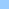# sumList

how to calculate the sum of a list of numbers
list sumsumList ```Q: I have a list of numbers. Is there an easy way to determine the sum of those numbers? may be something along theese lines will do? on enterFrame global theList set mylistSum = 0 set Cnt = count(theList) repeat with n = 1 to Cnt set myListSum = myListSum + getat(theList,n) end repeat if myListSum = 28 then go frame 40 else go the frame end if end ```Home shock + cgi Bits 'n pieces Director Lingo Shocklets Contact

pageID=l_sumList